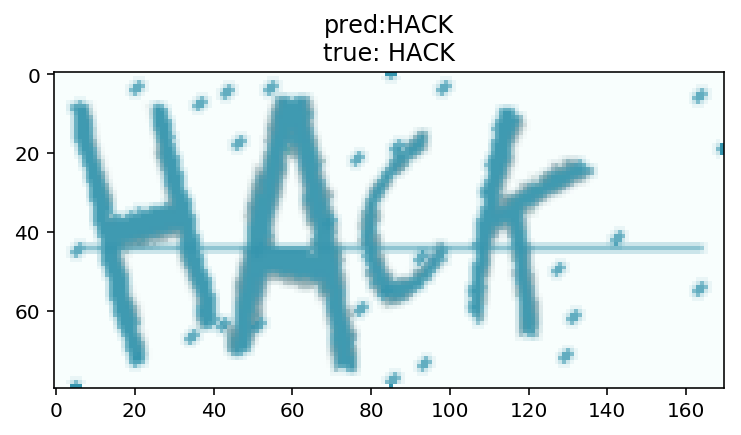GitHub 地址：github.com/ypwhs/captch

``````from captcha.image import ImageCaptcha
import matplotlib.pyplot as plt
import numpy as np
import random

%matplotlib inline
%config InlineBackend.figure_format = 'retina'

import string
characters = string.digits + string.ascii_uppercase
print(characters)

width, height, n_len, n_class = 170, 80, 4, len(characters)

random_str = ''.join([random.choice(characters) for j in range(4)])
img = generator.generate_image(random_str)

plt.imshow(img)
plt.title(random_str)
``````

# 数据生成器

## X

X 的形状是 (batch_size, height, width, 3)，比如一批生成32个样本，图片宽度为170，高度为80，那么形状就是 (32, 80, 170, 3)，取第一张图就是 X。

## y

y 的形状是四个 (batch_size, n_class)，如果转换成 numpy 的格式，则是 (n_len, batch_size, n_class)，比如一批生成32个样本，验证码的字符有36种，长度是4位，那么它的形状就是4个 (32, 36)，也可以说是 (4, 32, 36)，解码函数在下个代码块。

``````def gen(batch_size=32):
X = np.zeros((batch_size, height, width, 3), dtype=np.uint8)
y = [np.zeros((batch_size, n_class), dtype=np.uint8) for i in range(n_len)]
while True:
for i in range(batch_size):
random_str = ''.join([random.choice(characters) for j in range(4)])
X[i] = generator.generate_image(random_str)
for j, ch in enumerate(random_str):
y[j][i, :] = 0
y[j][i, characters.find(ch)] = 1
yield X, y
``````

# 使用生成器

``````def decode(y):
y = np.argmax(np.array(y), axis=2)[:,0]
return ''.join([characters[x] for x in y])

X, y = next(gen(1))
plt.imshow(X)
plt.title(decode(y))
``````

# 构建深度卷积神经网络

``````from keras.models import *
from keras.layers import *

input_tensor = Input((height, width, 3))
x = input_tensor
for i in range(4):
x = Convolution2D(32*2**i, 3, 3, activation='relu')(x)
x = Convolution2D(32*2**i, 3, 3, activation='relu')(x)
x = MaxPooling2D((2, 2))(x)

x = Flatten()(x)
x = Dropout(0.25)(x)
x = [Dense(n_class, activation='softmax', name='c%d'%(i+1))(x) for i in range(4)]
model = Model(input=input_tensor, output=x)

model.compile(loss='categorical_crossentropy',
metrics=['accuracy'])

``````

# 模型可视化

``````from keras.utils.visualize_util import plot
from IPython.display import Image

plot(model, to_file="model.png", show_shapes=True)
Image('model.png')
``````

``````brew install graphviz
pip install pydot-ng
``````

# 训练模型

``````model.fit_generator(gen(), samples_per_epoch=51200, nb_epoch=5,
nb_worker=2, pickle_safe=True,
validation_data=gen(), nb_val_samples=1280)
``````

# 测试模型

``````X, y = next(gen(1))
y_pred = model.predict(X)
plt.title('real: %s\npred:%s'%(decode(y), decode(y_pred)))
plt.imshow(X, cmap='gray')
``````

# 计算模型总体准确率

``````from tqdm import tqdm
def evaluate(model, batch_num=20):
batch_acc = 0
generator = gen()
for i in tqdm(range(batch_num)):
X, y = next(generator)
y_pred = model.predict(X)
y_pred = np.argmax(y_pred, axis=2).T
y_true = np.argmax(y, axis=2).T
batch_acc += np.mean(map(np.array_equal, y_true, y_pred))
return batch_acc / batch_num

evaluate(model)``````

# CTC Loss

• y_pred 是模型的输出，是按顺序输出的37个字符的概率，因为我们这里用到了循环神经网络，所以需要一个空白字符的概念；
• labels 是验证码，是四个数字；
• input_length 表示 y_pred 的长度，我们这里是15；
• label_length 表示 labels 的长度，我们这里是4。
``````from keras import backend as K

def ctc_lambda_func(args):
y_pred, labels, input_length, label_length = args
y_pred = y_pred[:, 2:, :]
return K.ctc_batch_cost(labels, y_pred, input_length, label_length)
``````

# 模型结构

``````from keras.models import *
from keras.layers import *
rnn_size = 128

input_tensor = Input((width, height, 3))
x = input_tensor
for i in range(3):
x = Convolution2D(32, 3, 3, activation='relu')(x)
x = Convolution2D(32, 3, 3, activation='relu')(x)
x = MaxPooling2D(pool_size=(2, 2))(x)

conv_shape = x.get_shape()
x = Reshape(target_shape=(int(conv_shape), int(conv_shape*conv_shape)))(x)

x = Dense(32, activation='relu')(x)

gru_1 = GRU(rnn_size, return_sequences=True, init='he_normal', name='gru1')(x)
gru_1b = GRU(rnn_size, return_sequences=True, go_backwards=True,
init='he_normal', name='gru1_b')(x)
gru1_merged = merge([gru_1, gru_1b], mode='sum')

gru_2 = GRU(rnn_size, return_sequences=True, init='he_normal', name='gru2')(gru1_merged)
gru_2b = GRU(rnn_size, return_sequences=True, go_backwards=True,
init='he_normal', name='gru2_b')(gru1_merged)
x = merge([gru_2, gru_2b], mode='concat')
x = Dropout(0.25)(x)
x = Dense(n_class, init='he_normal', activation='softmax')(x)
base_model = Model(input=input_tensor, output=x)

labels = Input(name='the_labels', shape=[n_len], dtype='float32')
input_length = Input(name='input_length', shape=, dtype='int64')
label_length = Input(name='label_length', shape=, dtype='int64')
loss_out = Lambda(ctc_lambda_func, output_shape=(1,),
name='ctc')([x, labels, input_length, label_length])

model = Model(input=[input_tensor, labels, input_length, label_length], output=[loss_out])
``````

# 数据生成器

``````def gen(batch_size=128):
X = np.zeros((batch_size, width, height, 3), dtype=np.uint8)
y = np.zeros((batch_size, n_len), dtype=np.uint8)
while True:
for i in range(batch_size):
random_str = ''.join([random.choice(characters) for j in range(4)])
X[i] = np.array(generator.generate_image(random_str)).transpose(1, 0, 2)
y[i] = [characters.find(x) for x in random_str]
yield [X, y, np.ones(batch_size)*int(conv_shape-2),
np.ones(batch_size)*n_len], np.ones(batch_size)
``````

# 评估模型

``````def evaluate(model, batch_num=10):
batch_acc = 0
generator = gen()
for i in range(batch_num):
[X_test, y_test, _, _], _  = next(generator)
y_pred = base_model.predict(X_test)
shape = y_pred[:,2:,:].shape
ctc_decode = K.ctc_decode(y_pred[:,2:,:],
input_length=np.ones(shape)*shape)
out = K.get_value(ctc_decode)[:, :4]
if out.shape == 4:
batch_acc += ((y_test == out).sum(axis=1) == 4).mean()
return batch_acc / batch_num
``````

# 评估回调

``````from keras.callbacks import *
class Evaluate(Callback):
def __init__(self):
self.accs = []

def on_epoch_end(self, epoch, logs=None):
acc = evaluate(base_model)*100
self.accs.append(acc)
print
print 'acc: %f%%'%acc
evaluator = Evaluate()
``````

# 训练模型

``````model.fit_generator(gen(), samples_per_epoch=51200, nb_epoch=100,
callbacks=[evaluator],
nb_worker=2, pickle_safe=True)
``````

# 测试模型

``````characters2 = characters + ' '
[X_test, y_test, _, _], _  = next(gen(1))
y_pred = base_model.predict(X_test)
y_pred = y_pred[:,2:,:]
out = K.get_value(K.ctc_decode(y_pred, input_length=np.ones(y_pred.shape)*y_pred.shape, ))[:, :4]
out = ''.join([characters[x] for x in out])
y_true = ''.join([characters[x] for x in y_test])

plt.imshow(X_test.transpose(1, 0, 2))
plt.title('pred:' + str(out) + '\ntrue: ' + str(y_true))

argmax = np.argmax(y_pred, axis=2)
list(zip(argmax, ''.join([characters2[x] for x in argmax])))
``````

# 有趣的问题

``````generator = ImageCaptcha(width=width, height=height)
random_str = 'O0O0'
X = generator.generate_image(random_str)
X = np.expand_dims(X, 0)

y_pred = model.predict(X)
plt.title('real: %s\npred:%s'%(random_str, decode(y_pred)))
plt.imshow(X, cmap='gray')
``````